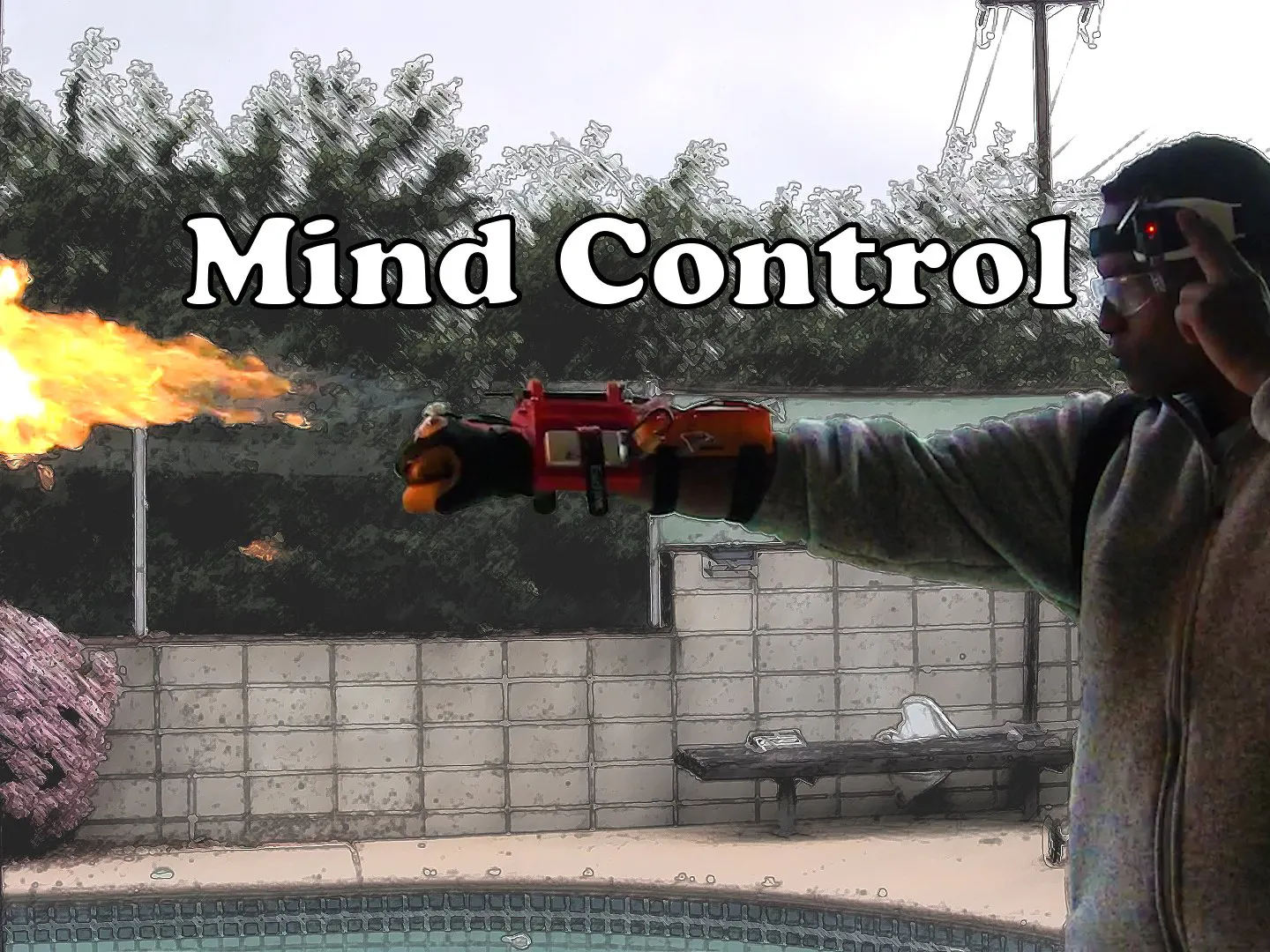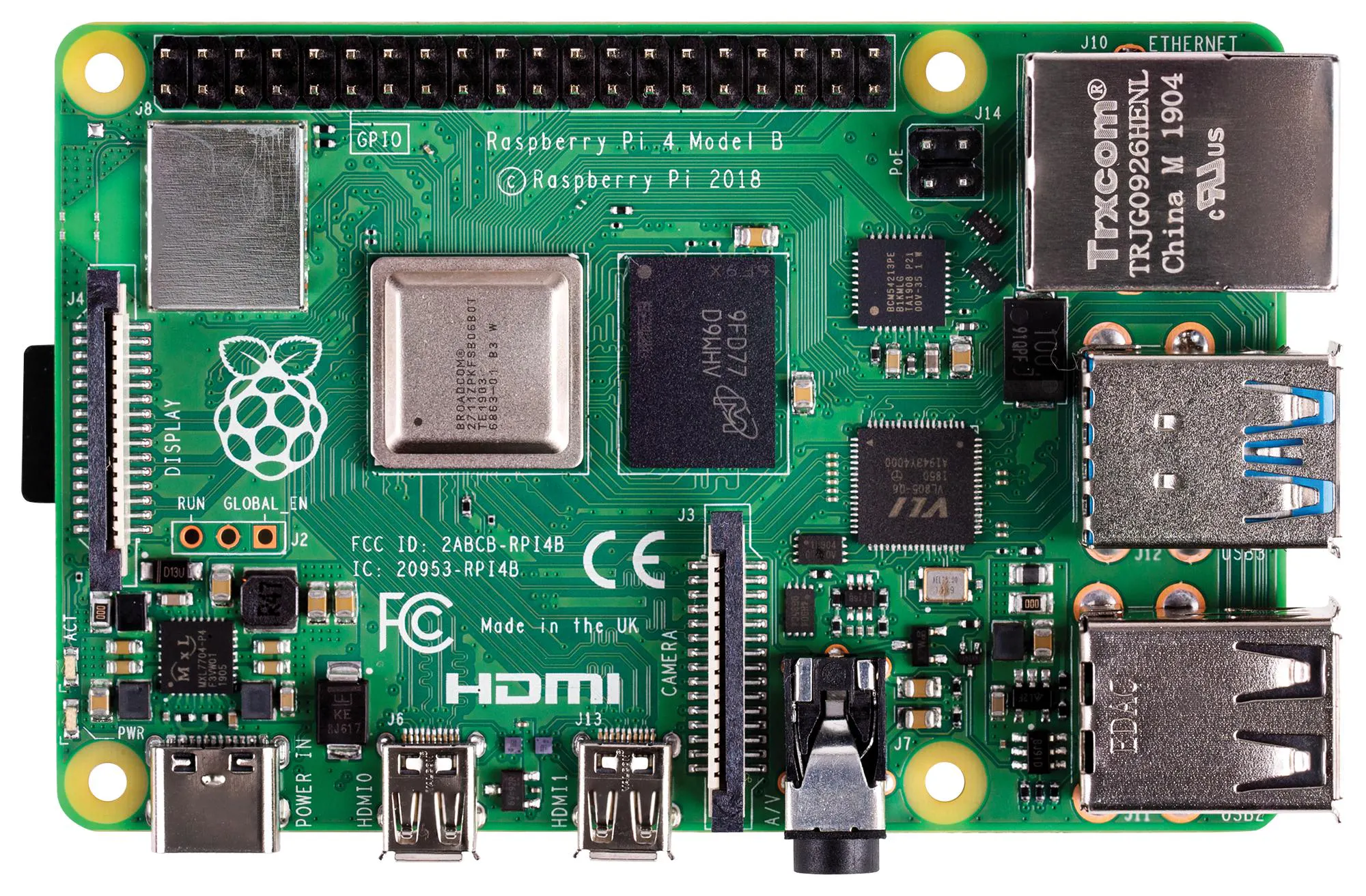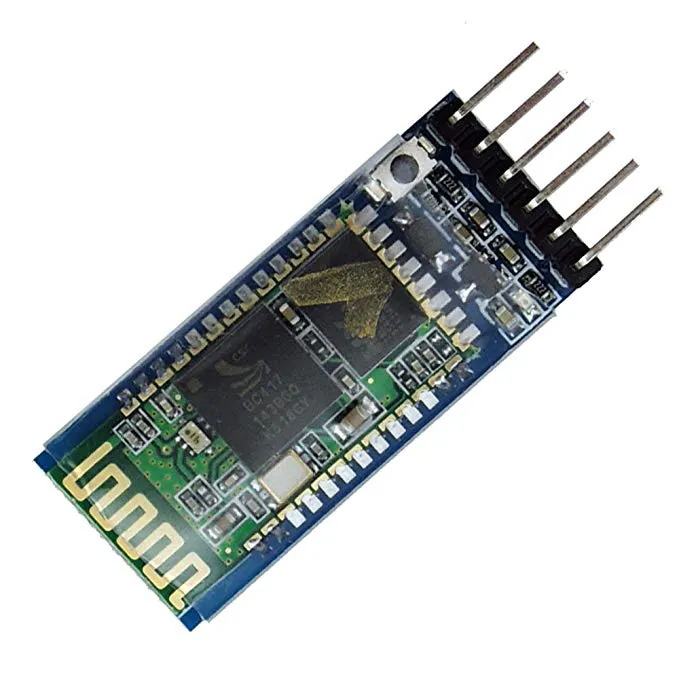# Using Machine Learning To Mind Control A Flamethrower

A mind controlled flamethrower that works off of thoughts of fire: no need to try to control your meditation or concentration values.## Things used in this project

### Hardware componentsArduino Nano R3
×2Raspberry Pi 4 Model B
×1HC-05 Bluetooth Module
×2

### Software apps and online servicesTensorFlowJupyter Notebook

### Hand tools and fabrication machines3D Printer (generic)Soldering iron (generic)

## Code

### brainfire.ino

Arduino
Code that runs the flamethrower
```#include <Wire.h>
#include <SPI.h>

#define SCREEN_WIDTH 128 // OLED display width, in pixels
#define SCREEN_HEIGHT 64 // OLED display height, in pixels

#define SolenoidPin 3
#define ArcLighterPin 6
//software SPI

unsigned long punchStart = 0;//variable for non-blocking punch timeframe check
unsigned long lastTimeAccelMeasured; // variable used to check how long the last acceleration value was checked

float lastAccelMeasured = 0;
const long punchInterval = 300;

int punchAccel = 50;//the beginning of a punch in m/s^2

int flameTime = 250;

int punchMode=0;
int mindMode =0;
int timeMode =1;

int sparkOn = 1;
int valveOn = 0;

const int leftButtonPin = 9;
const int rightButtonPin = 8;
const int upButtonPin = 10;
const int downButtonPin = 7;

const int mindInputPin = 2;
boolean buttonStateLeft = LOW;
boolean lastButtonStateLeft = LOW;
boolean currentButtonStateLeft = LOW;

boolean buttonStateRight = LOW;
boolean lastButtonStateRight = LOW;
boolean currentButtonStateRight = LOW;

boolean buttonStateUp = LOW;
boolean lastButtonStateUp = LOW;
boolean currentButtonStateUp = LOW;

boolean buttonStateDown = LOW;
boolean lastButtonStateDown = LOW;
boolean currentButtonStateDown = LOW;

unsigned long lastDebounceTime = 0;
unsigned long debounceDelay = 50;

void updateDisplayAllModes(){
display.clearDisplay();
display.display();
if(mindMode){
updateMindDisplay();
}
else if(punchMode){
updatePunchDisplay();
}
else if(timeMode){
updateTimeDisplay();
}
}

void Fire(int flameTime){
return;
}
if(valveOn){
digitalWrite(SolenoidPin, HIGH);
}
if(sparkOn){
digitalWrite(ArcLighterPin, LOW);
}
delay(flameTime);
digitalWrite(SolenoidPin, LOW);
digitalWrite(ArcLighterPin, HIGH);
if(mindMode){
}
delay(flameTime*2);
updateDisplayAllModes();
}

void updateMindDisplay(){
display.clearDisplay();
display.setCursor(0,0);
display.setTextColor(SSD1306_WHITE);
display.setTextSize(1.5);
display.setTextWrap(false);
display.print("Mind");

display.setCursor(0,20);
display.print("Valve:");
if(valveOn){
display.setCursor(40,20);
display.print("On");
}
else{
display.setCursor(40,20);
display.print("Off");
}

display.setCursor(0,40);
display.print("Spark:");
if(sparkOn){
display.setCursor(40,40);
display.print("On");
}
else{
display.setCursor(40,40);
display.print("Off");
}
display.setCursor(80,0);
display.setTextSize(8);
display.display();
}
void updatePunchDisplay(){
display.clearDisplay();
display.setCursor(0,0);
display.setTextColor(SSD1306_WHITE);
display.setTextSize(1.5);
display.setTextWrap(false);
display.print("Punch");

display.setCursor(0,20);
display.print("Valve:");
if(valveOn){
display.setCursor(40,20);
display.print("On");
}
else{
display.setCursor(40,20);
display.print("Off");
}

display.setCursor(0,40);
display.print("Spark:");
if(sparkOn){
display.setCursor(40,40);
display.print("On");
}
else{
display.setCursor(40,40);
display.print("Off");
}
display.setCursor(80,0);
display.setTextSize(8);
display.print("P");
display.display();

}

void updateTimeDisplay(){
display.clearDisplay();
display.setCursor(40,0);
display.setTextSize(2);
display.setTextColor(SSD1306_WHITE);
display.print("Time");

int16_t x1, y1;
uint16_t w, h;

display.setTextSize(4);
display.getTextBounds(String(flameTime), 0, 0, &x1, &y1, &w, &h);
display.setCursor(64 -(w/2) ,32);
display.setTextColor(SSD1306_WHITE);
display.print(String(flameTime));
//display.drawLine(64,0,64,64,SSD1306_WHITE);
display.display();
}
void upButtonPress(){
if(mindMode){
}
updateDisplayAllModes();
delay(1000);
}
else if(punchMode){
}
else if(timeMode){
if(flameTime<1000){
flameTime +=10;
}
updateDisplayAllModes();

}

}
void downButtonPress(){
if(mindMode){
}
}
else if(punchMode){
}
else if(timeMode){
if(flameTime>10){
flameTime -=10;
}

}
updateDisplayAllModes();
}
void leftButtonPress(){
if(mindMode || punchMode){
if(valveOn && sparkOn){
sparkOn = 0;
valveOn = 1;
}
else if(!valveOn && !sparkOn){
valveOn =0;
sparkOn =1;
}
else if(valveOn){
valveOn = 0;
sparkOn = 0;
}
else if(sparkOn){
valveOn = 1;
sparkOn = 1;
}
updateDisplayAllModes();
}

}
void rightButtonPress(){
if(mindMode){
mindMode = 0;
punchMode = 1;
timeMode = 0;
updateDisplayAllModes();
}
else if(punchMode){
mindMode = 0;
punchMode = 0;
timeMode = 1;
updateDisplayAllModes();
}
else if(timeMode){
mindMode = 1;
punchMode = 0;
timeMode = 0;
updateDisplayAllModes();
}
}
void setup(void) {
//Test to see if accelerometer is communicating
Serial.begin(9600);
Serial.println("LIS3DH test!");
if (! lis.begin(0x18) ) {   // change this to 0x19 for alternative i2c address
Serial.println("Couldnt start");
while (1);
}
Serial.println(F("SSD1306 allocation failed"));
for(;;); // Don't proceed, loop forever
}

//display.clearDisplay();
//display.display();
Serial.println("LIS3DH found!");

lis.setRange(LIS3DH_RANGE_16_G);   //+-16G range for good punch detection
Serial.print("Range = "); Serial.print(2 << lis.getRange());
Serial.println("G");

pinMode(SolenoidPin, OUTPUT);
pinMode(ArcLighterPin, OUTPUT);

pinMode(leftButtonPin, INPUT_PULLUP);
pinMode(rightButtonPin, INPUT_PULLUP);
pinMode(upButtonPin, INPUT_PULLUP);
pinMode(downButtonPin, INPUT_PULLUP);

pinMode(mindInputPin, INPUT);

digitalWrite(SolenoidPin, LOW);
digitalWrite(ArcLighterPin, HIGH);

lastTimeAccelMeasured = millis();
updateDisplayAllModes();
delay(500);
}

void loop() {
if(mindMode){
Serial.println((String)"Punch:" +(String)flameTime);
Fire(flameTime);
}
}
else if(punchMode){

sensors_event_t event;
lis.getEvent(&event);

//look for punch
unsigned long currentMillis = millis();
float currentAccel = event.acceleration.x;

if (event.acceleration.y > punchAccel){
Serial.println(event.acceleration.y);

Serial.println((String)"Punch:" +(String)flameTime);
Fire(flameTime);
}
}
else if(timeMode){

}

if (currentButtonStateLeft != lastButtonStateLeft || currentButtonStateRight != lastButtonStateRight ||
currentButtonStateUp != lastButtonStateUp || currentButtonStateDown != lastButtonStateDown)

{
lastDebounceTime = millis();
}

if ((millis() - lastDebounceTime) > debounceDelay) {
if (currentButtonStateLeft != buttonStateLeft)
{
buttonStateLeft = currentButtonStateLeft;
Serial.println("left okay");
if (buttonStateLeft == LOW)
{
leftButtonPress();
}

}
if (currentButtonStateRight != buttonStateRight)
{
buttonStateRight = currentButtonStateRight;

if (buttonStateRight == LOW)
{
rightButtonPress();
}

}
if (currentButtonStateUp != buttonStateUp)
{
buttonStateUp = currentButtonStateUp;

if (buttonStateUp == LOW)
{
upButtonPress();
}

}
if (currentButtonStateDown != buttonStateDown)
{
buttonStateDown = currentButtonStateDown;

if (buttonStateDown == LOW)
{
downButtonPress();
}

}

}
lastButtonStateLeft = currentButtonStateLeft;
lastButtonStateRight = currentButtonStateRight;
lastButtonStateUp = currentButtonStateUp;
lastButtonStateDown = currentButtonStateDown;

}

```

### mind.py

Python
Used to run inference on the model
```import sys
import serial
import time
import numpy as np
import tflite_runtime.interpreter as lite
from pathlib import Path
#import pyeeg
import RPi.GPIO as GPIO

ser = serial.Serial('/dev/ttyS0', 57600)

samplingRate = 512

bands = [4, 7, 12, 30, 100]

EEGVALUES = []

rawData = []

windowLength = 256

maxPacketLength = 169
GPIO.setmode(GPIO.BOARD)
GPIO.setup(32, GPIO.OUT)

interpreter = lite.Interpreter(model_path="model.tflite")
interpreter.allocate_tensors()

input_details = interpreter.get_input_details()
output_details = interpreter.get_output_details()

print(input_details)

print(output_details)

POOR_SIGNAL = 0

def updateRaw(raw):
global rawData
global bands
global samplingRate

if (float(raw) > 1000.0):
rawData.append(1.0)
elif (float(raw) < -1000.0):
rawData.append(-1.0)
else:
rawData.append(float(raw)/1000)

if(len(rawData) >= windowLength):
ser.reset_input_buffer()
rawData = np.reshape(np.array(rawData, dtype=np.float32), (1, 256, 1))

# fft = pyeeg.bin_power(rawData, bands, samplingRate)/20000
interpreter.set_tensor(input_details['index'], rawData)
interpreter.invoke()
output_data = interpreter.get_tensor(output_details['index'])
if(output_data > 0.7):

GPIO.output(32, GPIO.HIGH)

print("fire")
else:
GPIO.output(32, GPIO.LOW)
rawData = []
ser.reset_input_buffer()

callback = updateRaw

def setCallback(callbackFunction):
callback = callbackFunction

def parsePacket(packet):
i = 1
while i < len(packet) - 1:

if ord(packet[i]) == 0x02:
POOR_SIGNAL = ord(packet[i+1])
# print(POOR_SIGNAL)
i += 2
elif ord(packet[i]) == 0x04:
ATTENTION = ord(packet[i+1])
i += 2
elif ord(packet[i]) == 0x05:
MEDITATION = ord(packet[i+1])
i += 2
elif ord(packet[i]) == 0x16:
i += 2
elif ord(packet[i]) == 0x83:
# for c in xrange(i+1, i+25, 3):
#EEGVALUES.append(ord(packet[c]) << 16 | ord(packet[c+1]) << 8 | ord(packet[c+2]))
i += 26
elif ord(packet[i]) == 0x80:
raw = ord(packet[i+2])*256 + ord(packet[i+3])
if(raw >= 32768):
raw = raw - 65536

callback(raw)

i += 4

return True

previousByte = 'c'
inPacket = False
packet = []

while True:

# print(ord(previousByte))
# print(ord(currentByte))
if(not inPacket and ord(previousByte) == 224 and ord(currentByte) == 224):
bytearay = b'\x00\xF8\x00\x00\x00\xE0'
ser.write(bytearay)
time.sleep(1)
continue
# checks for start of packet in two syncs
if (not inPacket and ord(previousByte) == 170 and ord(currentByte) == 170):
inPacket = True

packet = []
continue

elif inPacket:
if len(packet) == 0:
if ord(currentByte) == 170:
continue

# first packet after syncs is packet length
packetLength = ord(currentByte)
totalChecksum = 0
packet = [currentByte]
if packetLength > maxPacketLength:
inPacket = False
continue

elif len(packet) - 1 == packetLength:  # checking to see if at end of packet
packetChecksum = ord(currentByte)
inPacket = False
if (~(totalChecksum & 255) & 255) == packetChecksum:
# print("hello")
parsePacket(packet)
# else:
#print("bruh error")

else:
totalChecksum += ord(currentByte)
packet.append(currentByte)
previousByte = currentByte

ser.reset_input_buffer()
```

### data.py

Python
Used to collect the mind data
```import os
import sys
import serial
import time
import numpy as np
#from biosppy import storage
import datetime

ser = serial.Serial('/dev/ttyS0', 57600)

EEGVALUES = []

rawData = []

maxPacketLength = 169
recordingTime = 1

timeNow = datetime.datetime.now()
timeNow = timeNow.strftime("%m-%d-%Y-%H-%M-%S")

standard = True

POOR_SIGNAL = 0

def updateRaw(raw):
global recordingTime

rawData.append([raw])

print(len(rawData))
if(len(rawData) > recordingTime*60*512):
if(standard):
pathlib = os.path.join(os.path.expanduser("~"), "/data/standard")
path = os.path.join(pathlib, timeNow + '.txt')
f = open(path, "w+")
np.savetxt(f, rawData)
f.close()
else:
pathlib = os.path.join(os.path.expanduser("~"), "/data/fire")
path = os.path.join(pathlib, timeNow + '.txt')
f = open(path, "w+")
np.savetxt(f, rawData)
f.close()

sys.exit()

callback = updateRaw

def setCallback(callbackFunction):
callback = callbackFunction

def parsePacket(packet):
i = 1
while i < len(packet) - 1:

if ord(packet[i]) == 0x02:
POOR_SIGNAL = ord(packet[i+1])
print(POOR_SIGNAL)
i += 2
elif ord(packet[i]) == 0x04:
ATTENTION = ord(packet[i+1])
i += 2
elif ord(packet[i]) == 0x05:
MEDITATION = ord(packet[i+1])
i += 2
elif ord(packet[i]) == 0x16:
i += 2
elif ord(packet[i]) == 0x83:
# for c in xrange(i+1, i+25, 3):
#EEGVALUES.append(ord(packet[c]) << 16 | ord(packet[c+1]) << 8 | ord(packet[c+2]))
i += 26
elif ord(packet[i]) == 0x80:
raw = ord(packet[i+2])*256 + ord(packet[i+3])
if(raw >= 32768):
raw = raw - 65536

callback(raw)

i += 4

return True

previousByte = 'c'
inPacket = False
packet = []

while True:

# print(ord(previousByte))
# print(ord(currentByte))
if(not inPacket and ord(previousByte) == 224 and ord(currentByte) == 224):
ser.write("This is a test".encode())
# time.sleep(1)
continue
# checks for start of packet in two syncs
if (not inPacket and ord(previousByte) == 170 and ord(currentByte) == 170):
inPacket = True

packet = []
continue

elif inPacket:
if len(packet) == 0:
if ord(currentByte) == 170:
continue

# first packet after syncs is packet length
packetLength = ord(currentByte)
totalChecksum = 0
packet = [currentByte]
if packetLength > maxPacketLength:
inPacket = False
continue

elif len(packet) - 1 == packetLength:  # checking to see if at end of packet
packetChecksum = ord(currentByte)
inPacket = False
if (~(totalChecksum & 255) & 255) == packetChecksum:
# print("hello")
parsePacket(packet)
else:
print("callback error")

else:
totalChecksum += ord(currentByte)
packet.append(currentByte)
previousByte = currentByte

print("type f for fire or s for standard")
x = input()
if(x == 'f'):
print("doing fire")
standard = False
elif(x == 's'):
standard = True
print("doing standard")

print("type number of minutes wanted")

x = input()

recordingTime = float(x)
ser.reset_input_buffer()
```

### 0x02.ino

Arduino
Used to convert the baudrate of the mindflex
```void setup() {
// Open serial communications and wait for port to open:
Serial.begin(9600);

delay(1000);
Serial.write(0x02);
}

void loop() { // run over and over

}
```

### Jupyter Lab

Used to train the model

## Credits

### Nathaniel Felleke

5 projects • 34 followers
Developer always trying to make new things. MIT Class of 2026. @EdgeImpulse Expert.